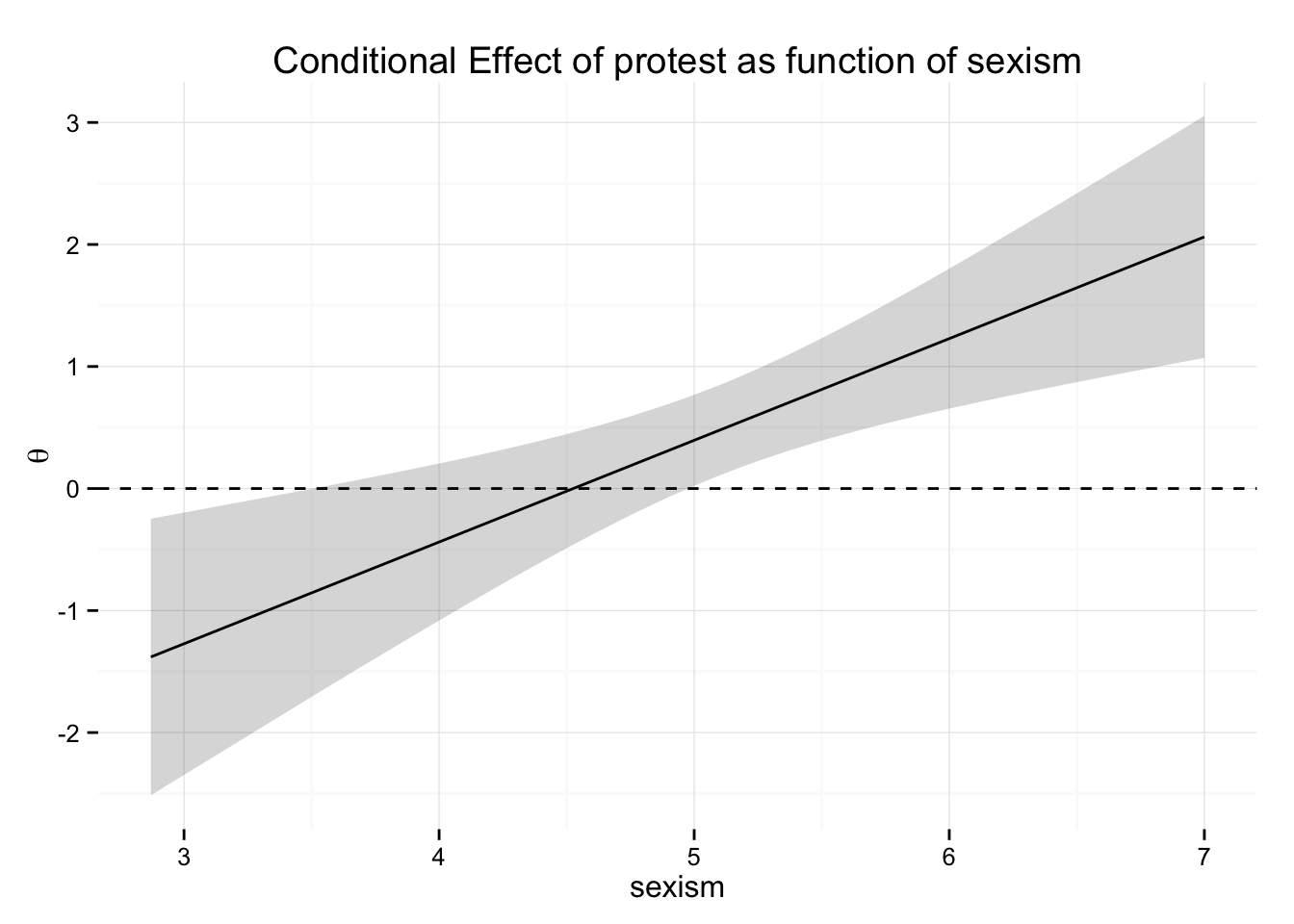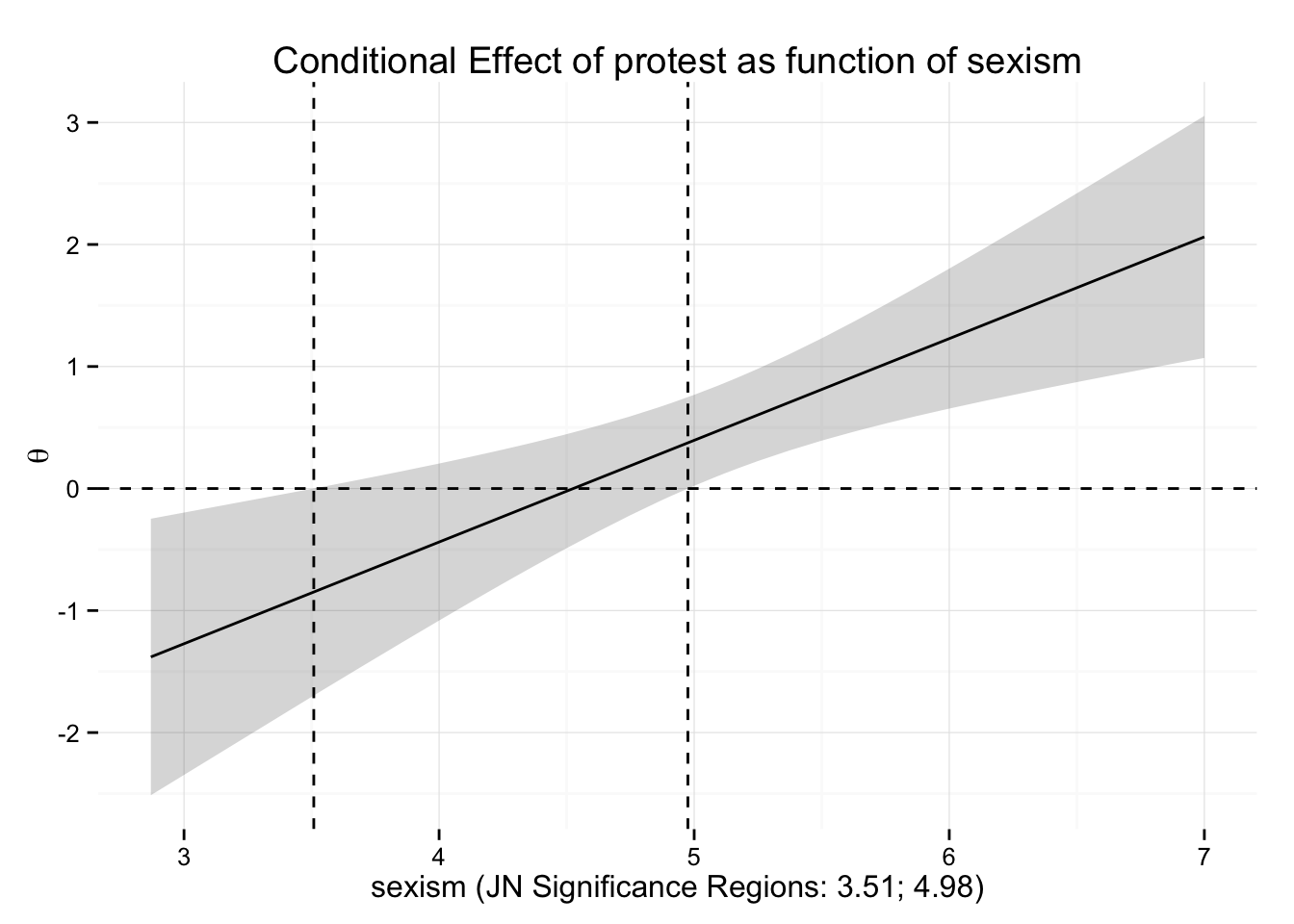### Motivation

Moderated effects or interaction effects in regression-type models are best understood by plotting the effects of interest as a function of the moderator. Andrew Hayes (2013, p. 242) described a plot of the conditional effect $$\theta$$ of a focal predictor X across the values of a moderator Z. His PROCESS macro provides some helpful output to create such a plot in SPSS. However, creating this plot (or almost any other plot, really) in SPSS in no fun — even less so if you plan to format the plot in some kind of publication-ready style. This was the reason why I wrote a function to replicate the conditional effect plot in R. While I was at it, I thought it would be nice to implement the Johnson‐Neyman Technique (JNT). The JNT makes it easy to probe $$\theta$$ across all values of Z as recommended in the literature (see for example Bauer & Curran or Brambor et al.).

### Limitations and warning

The functions described in the following were neither thoroughly tested nor implemented with great care. I document them here for myself and others who might find them useful. The results might be wrong and the functions may contain several bugs. Don’t trust them.

### Dataset for demonstration

The data come from a study by Garcia et al. and were made available as demo data by Hayes as part of his PROCESS macro. The moderation analysis of this data set is described in great detail in Hayes’ Chapter 7.

require(foreign)
require(dplyr)
protest = tbl_df(read.spss("3 protest.sav", use.value.labels = F, to.data.frame = T))
protest
## Source: local data frame [129 x 5]
##
##    sexism liking respappr protest x
## 1    4.87   4.83     4.25       1 3
## 2    4.25   4.50     5.75       0 4
## 3    5.00   5.50     4.75       1 4
## 4    5.50   5.66     7.00       1 3
## 5    5.62   6.16     6.75       1 3
## 6    5.75   6.00     5.50       1 4
## 7    5.12   4.66     5.00       1 3
## 8    6.62   6.50     6.25       0 5
## 9    5.75   1.00     3.00       0 2
## 10   4.62   6.83     5.75       0 6
## ..    ...    ...      ...     ... .

protest is the focal predictor X, sexism is the moderator Z, and liking is the dependent variable Y.

protest.lm = lm(liking ~ protest * sexism, protest)
summary(protest.lm)
##
## Call:
## lm(formula = liking ~ protest * sexism, data = protest)
##
## Residuals:
##     Min      1Q  Median      3Q     Max
## -3.9894 -0.6381  0.0478  0.7404  2.3650
##
## Coefficients:
##                Estimate Std. Error t value Pr(>|t|)
## (Intercept)      7.7062     1.0449   7.375 1.99e-11 ***
## protest         -3.7727     1.2541  -3.008  0.00318 **
## sexism          -0.4725     0.2038  -2.318  0.02205 *
## protest:sexism   0.8336     0.2436   3.422  0.00084 ***
## ---
## Signif. codes:  0 '***' 0.001 '**' 0.01 '*' 0.05 '.' 0.1 ' ' 1
##
## Residual standard error: 0.9888 on 125 degrees of freedom
## Multiple R-squared:  0.1335, Adjusted R-squared:  0.1127
## F-statistic: 6.419 on 3 and 125 DF,  p-value: 0.0004439

The significant interaction protest:sexism shows that the effect of X on Y is moderated by Z.

### Function 1: Implementing the Johnson‐Neyman Technique

jnt = function(.lm, predictor, moderator, alpha=.05) {
require(stringi)
b1 = coef(.lm)[predictor]
b3 = coef(.lm)[stri_startswith_fixed(names(coef(.lm)), paste0(predictor,":")) | stri_endswith_fixed(names(coef(.lm)), paste0(":",predictor))]
se_b1 = coef(summary(.lm))[predictor, 2]
se_b3 = coef(summary(.lm))[stri_startswith_fixed(names(coef(.lm)), paste0(predictor,":")) | stri_endswith_fixed(names(coef(.lm)), paste0(":",predictor)), 2]
COV_b1b3 = vcov(.lm)[predictor, stri_startswith_fixed(names(coef(.lm)), paste0(predictor,":")) | stri_endswith_fixed(names(coef(.lm)), paste0(":",predictor))]
t_crit = qt(1-alpha/2, .lm$df.residual) # see Bauer & Curran, 2005 a = t_crit^2 * se_b3^2 - b3^2 b = 2 * (t_crit^2 * COV_b1b3 - b1 * b3) c = t_crit^2 * se_b1^2 - b1^2 jn = c( (-b - sqrt(b^2 - 4 * a * c)) / (2 * a), (-b + sqrt(b^2 - 4 * a * c)) / (2 * a) ) JN = sort(unname(jn)) JN = JN[JN>=min(.lm$model[,moderator]) & JN<=max(.lm$model[,moderator])] JN } The function could probably be written more elegantly, but it works for my purpose. The computations required for the JNT are for example described by Andrew Hayes or Bauer & Curran. I will not go into detail here. jnt() requires three inputs: • .lm is the fitted linear model which includes an interaction (The function also works for glm, but the interpretation of interactions in such models is much more complicated than one would assume). • predictor gives the variable name of X, moderator gives the variable name of Z. The names must exactly match the names in the data set! • Additionally, alpha specifies the desired level of significance. The default value is .05. jnt(protest.lm, predictor = "protest", moderator = "sexism", alpha = .05) ##  3.508712 4.975297 The function returns a numeric vector of length 1, 2, or 0. The values are the boundaries of the JN regions of significance. ### Function 2: Plotting $$\theta$$ as a function of Z theta_plot = function(.lm, predictor, moderator, alpha=.05, jn=F) { require(dplyr) require(ggplot2); theme_set(theme_minimal()) require(stringi) .data = data_frame(b1 = coef(.lm)[predictor], b3 = coef(.lm)[stri_startswith_fixed(names(coef(.lm)), paste0(predictor,":")) | stri_endswith_fixed(names(coef(.lm)), paste0(":",predictor))], Z = quantile(.lm$model[,moderator], seq(0,1,.01)),
theta = b1 + Z * b3,
se_b1 = coef(summary(.lm))[predictor, 2],
COV_b1b3 = vcov(.lm)[predictor, stri_startswith_fixed(names(coef(.lm)), paste0(predictor,":")) | stri_endswith_fixed(names(coef(.lm)), paste0(":",predictor))],
se_b3 = coef(summary(.lm))[stri_startswith_fixed(names(coef(.lm)), paste0(predictor,":")) | stri_endswith_fixed(names(coef(.lm)), paste0(":",predictor)), 2],
se_theta = sqrt(se_b1^2 + 2 * Z * COV_b1b3 + Z^2 * se_b3^2),
ci.lo_theta = theta+qt(alpha/2, .lm$df.residual)*se_theta, ci.hi_theta = theta+qt(1-alpha/2, .lm$df.residual)*se_theta)
if (jn) {
JN = jnt(.lm=.lm, predictor=predictor, moderator=moderator, alpha=alpha)
JN_lines = geom_vline(xintercept=JN, linetype=2)
JN_regions = ifelse(length(JN) == 0, "no significance regions", paste(round(JN,2), collapse = "; "))
Xlab = paste0(moderator, " (JN Significance Regions: ", JN_regions,")")
}
else {
Xlab = moderator
JN_lines = NULL
}
.data %>%
ggplot(aes(Z, theta, ymin=ci.lo_theta, ymax=ci.hi_theta)) + geom_ribbon(alpha = .2) + geom_line() + ggtitle(paste("Conditional Effect of", predictor, "as function of", moderator)) + geom_hline(yintercept=0, linetype=2) + labs(x = Xlab, y=expression(theta)) + JN_lines
}

theta_plot() requires the same three inputs as jnt():

• .lm is the fitted linear model which includes an interaction (The function also works for glm, but the interpretation of interaction in such models is much more complicated than one would assume.)
• predictor gives the variable name of X, moderator gives the variable name of Z. The names must exactly match the names in the data set!
• alpha specifies the desired level of significance. The default value is .05.
• Additionally, jn indicates whether the JN significance regions should be added to the plot. This is done by calling jnt().
theta_plot(protest.lm, predictor = "protest", moderator = "sexism", alpha = .05, jn = F)The plot shows that the effect of X on Y is (significantly) negative for people low in Z and (significantly) positive for people high in Z. With the help of the JNT we can specify more in detail for which people significant effects can be found.

tmp_plot = theta_plot(protest.lm, predictor = "protest", moderator = "sexism", alpha = .05, jn = T)
class(tmp_plot)
##  "gg"     "ggplot"
tmp_plottheta_plot() returns a ggplot object. It can be easily edited to achieve publication-ready results (not shown here ;).